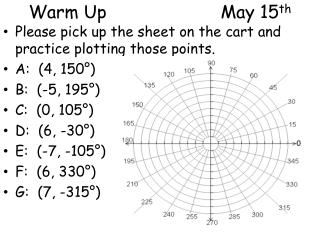Download PresentationWarm Up May 15 thWarm Up May 15 th - PowerPoint PPT Presentation

Download PresentationWarm Up May 15 th
An Image/Link below is provided (as is) to download presentation

Download Policy: Content on the Website is provided to you AS IS for your information and personal use and may not be sold / licensed / shared on other websites without getting consent from its author. While downloading, if for some reason you are not able to download a presentation, the publisher may have deleted the file from their server.

- - - - - - - - - - - - - - - - - - - - - - - - - - - E N D - - - - - - - - - - - - - - - - - - - - - - - - - - -
Presentation Transcript

1. Warm Up May 15th • Please pick up the sheet on the cart and practice plotting those points. • A: (4, 150°) • B: (-5, 195°) • C: (0, 105°) • D: (6, -30°) • E: (-7, -105°) • F: (6, 330°) • G: (7, -315°)

2. Homework Check/Questions #1 *FLOWER*

3. Homework Check/Questions #2 *HEART OR APPLE*

4. Polar Graphing Converting Points & Equations between polar and rectangular

5. “Equivalent” Points… • Which of the following polar coordinate pairs represent the same point as the point with polar coordinates (2, 105º)? • (2, -75º) • (-2, -75º) • (-2, -105º) • (-2, -255º) • (-2, 285º)

6. Point conversion… • Convert the point with polar coordinates to rectangular coordinates… x = rcos  y = rsin 

7. Point conversion… • Convert the point with rectangular coordinates to polar coordinates… r2 = x2 + y2 tan  = y/x (then put  in the correct quadrant)

8. Practice… NO Calculator… • Convert (-3, -3) to polar coordinates. • Convert (6, π) to rectangular coordinates. • Convert (-4, 2π/3) to rectangular. • Calculator Active… (round to 3 decimal places) • Convert (-2, 5) to polar coordinates. • Convert (10, 172º) to rectangular coordinates.

9. Equation ConversionPolar to Rectangular *It’s all about substitution* Examples: • r = 3 •  = /4 • r = csc  • r = 2cos 

10. Equation ConversionRectangular to Polar *It’s all about substitution* Examples • y = 2 • x2 + y2 = 25 • 2x + 3y = -3 • x2 + y2 - 8x = 0

11. Practice Convert the equations from polar to rectangular. Convert the equations from rectangular to polar. 3) x2 – 3y = 0 4) 5x - y = 1